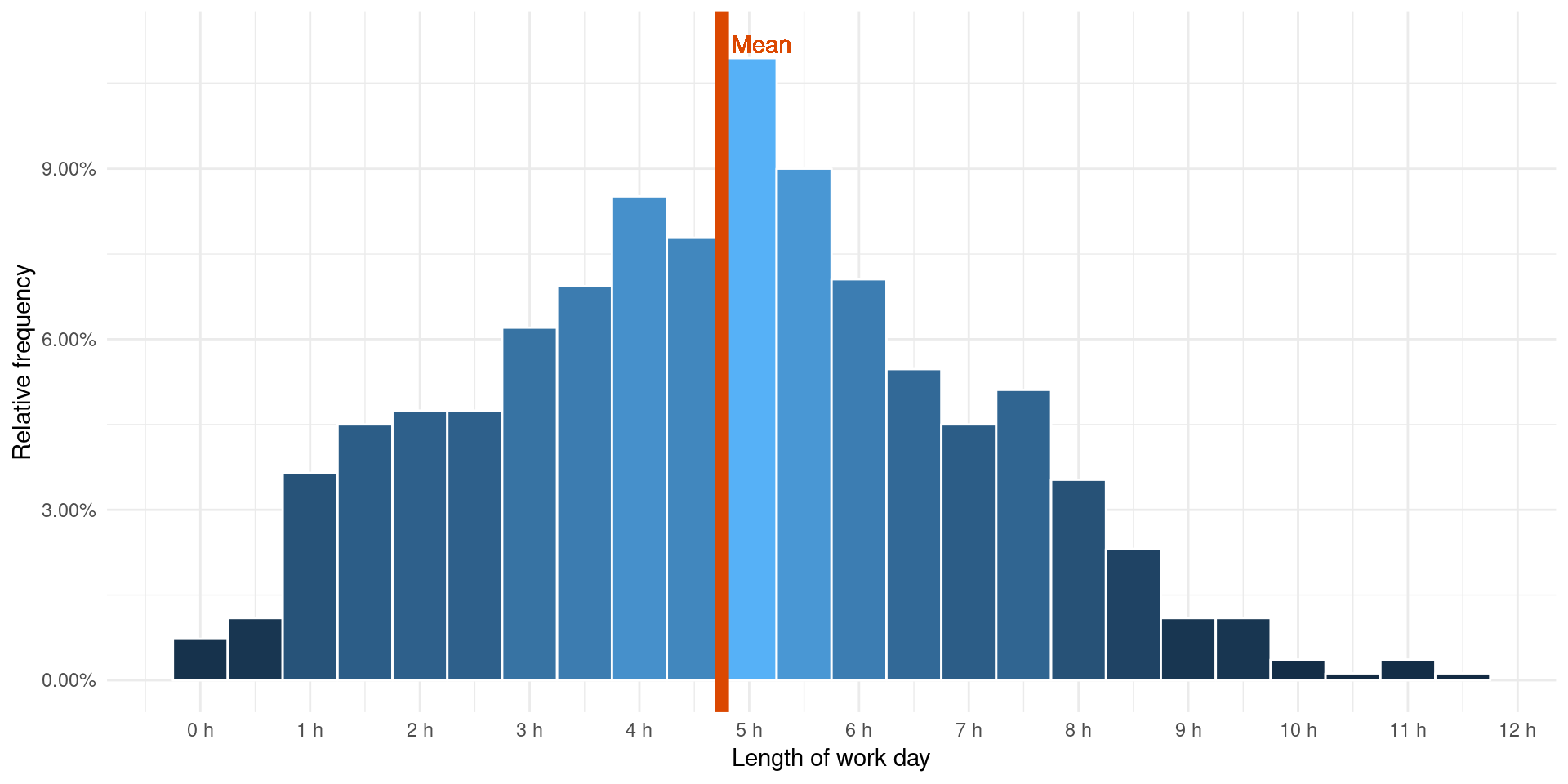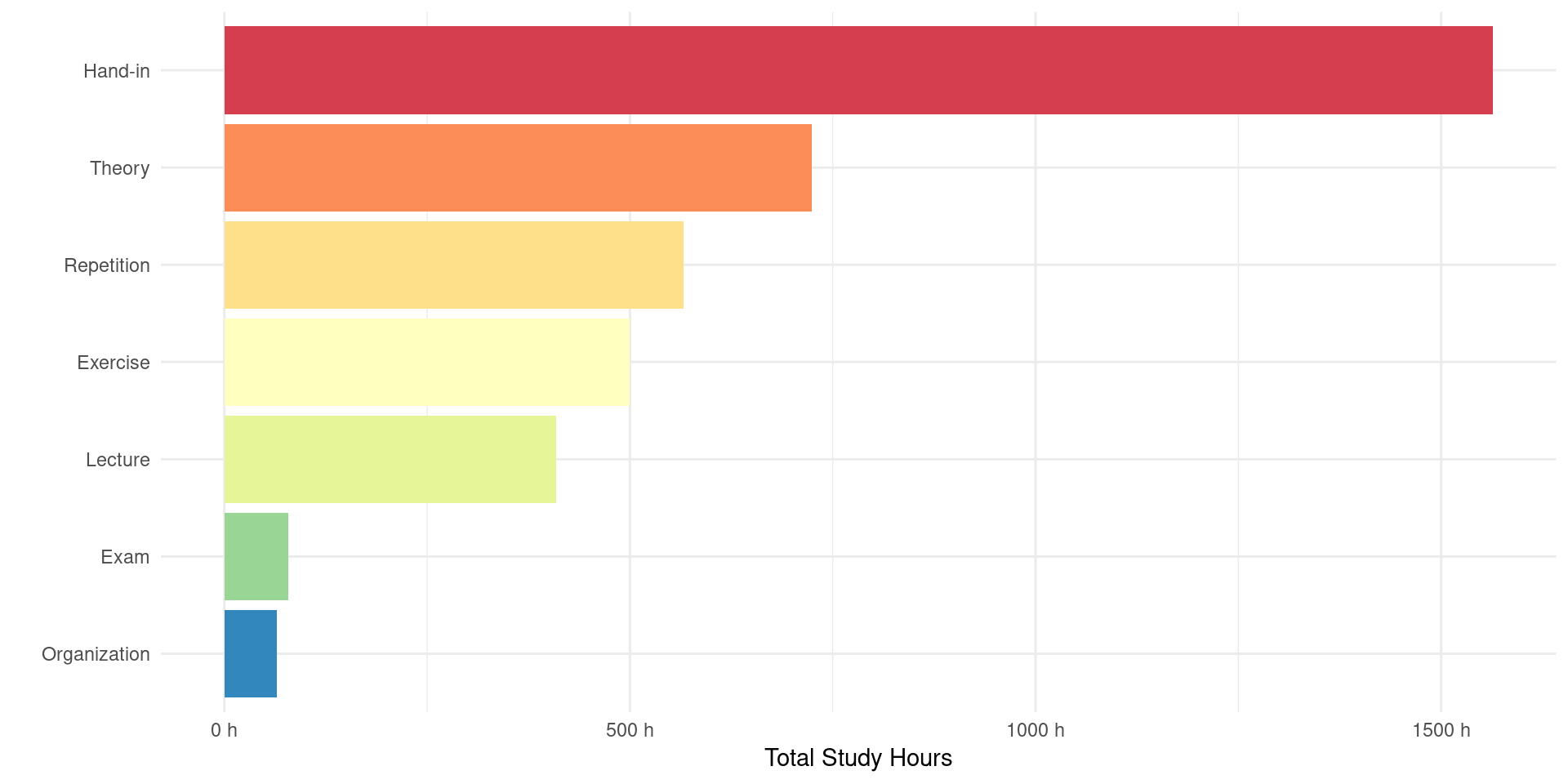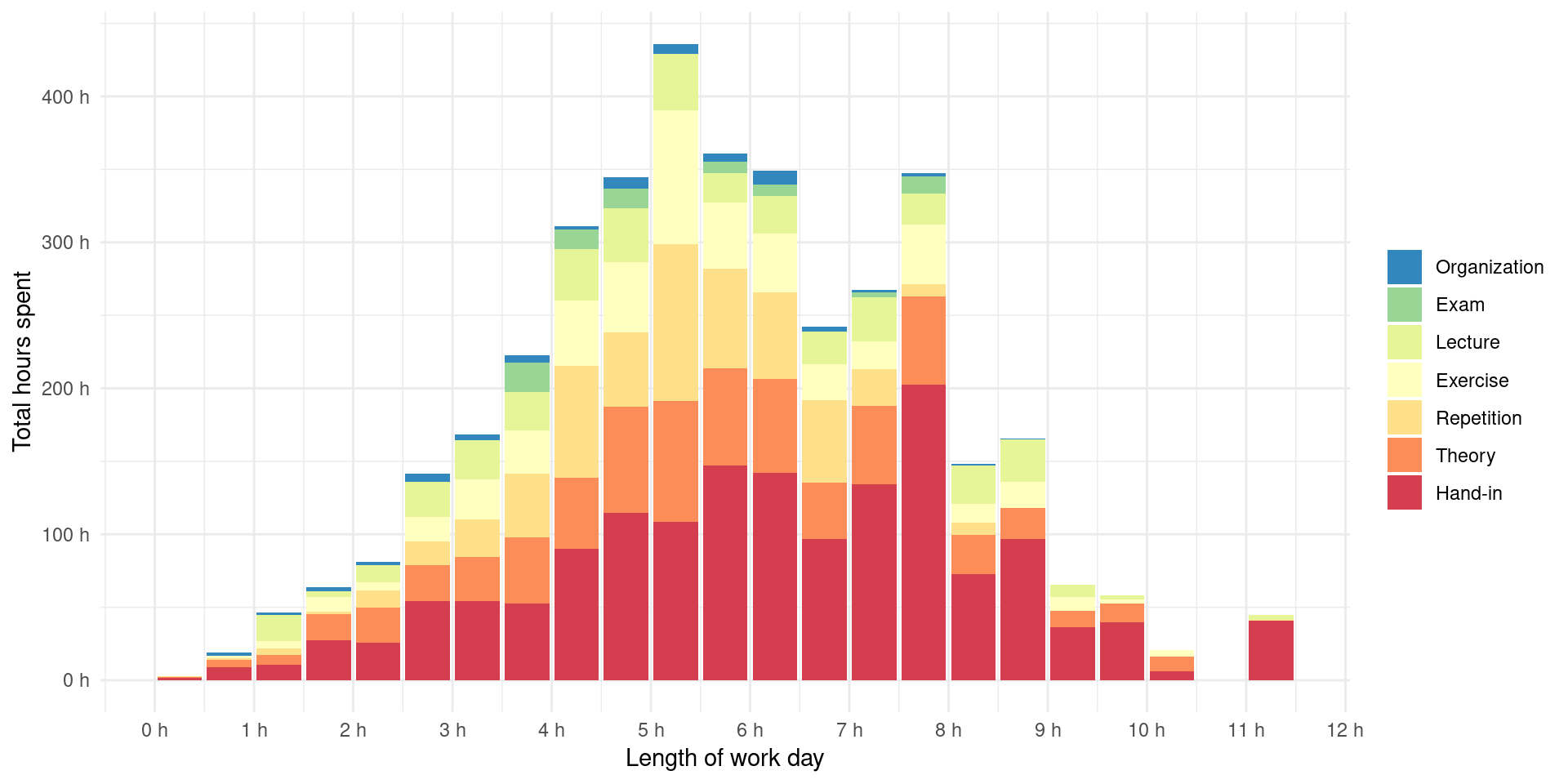``````# --- All the R-libraries used for this analysis ---

# Ecosystem of modern R-packages
library(tidyverse)

# For parsing JSON
library(tidyjson)

# For reshaping data frames, e.g. `spread`
library(reshape2)

# For `rollmean`
library(zoo)

# For outputting dataframes as HTML tables
library(kableExtra)

# For use of `percent_format()`
library(scales)

# For custom color schemes in ggplot
library(RColorBrewer)

# Alternative JSON parsing library
library(jsonlite)

# For `lookup_by_column`
library(rqdatatable)

# For various datetime functionality
library(lubridate)

# For assignment pipe functionality
library(magrittr)``````

# Introduction

A master’s degree is a 5 year long endeavour that often is summarized in a measly, one-page grade sheet. That feels quite unsatisfying as there are so many aspects of an education that ends up being entirely neglected. As a student that is nearing the end of my education in “Applied Physics and Mathematics” with a specialization in statistics at NTNU I want to rectify this problem

I have been tracking my time usage as a student since I started at the university in August 2014. Every time I sat down with study related work I started a Toggl timer. If I have to go to the bathroom, talk with another student, etc., I stop the timer even if it is for only two minutes. It is therefore intended that the data set can accurately reflect the number of effective hours I have spent studying for the last 5 years. I hope this may prove insightful for other students, professors wondering what their students are doing, and (hopefully) prospective employers.

I have written a small python library for requesting/scraping data from various sources such as Toggl, Runkeeper, SleepCycle, Sleep as Android, and grades.no. It is this data we will sanitize, visualize, and analyze using R in this article. At any point you can press the “Code” button on the right hand side if you wonder how the data is visualized.

The Toggl time entries end up looking like this:

``````# Reading JSON generated by python library

# Parse the JSON structure into a tidy data frame
toggl <- toggl_json %>%
as.tbl_json() %>%
gather_array() %>%
description = jstring("description"),
date = jstring("start"),
start = jstring("start"),
end = jstring("end"),
study_session_duration = jnumber("dur"),
project = jstring("project")
) %>%
mutate(
date = date(date),
start = ymd_hms(start),
end = ymd_hms(end),
study_session_duration = study_session_duration / (1000 * 60 * 60)
) %>%
rename(course = "project") %>%
dplyr::filter(study_session_duration < 12) %>%
select(-document.id, -array.index)

# Sanitize the descriptions into 8 main categories
toggl\$description %<>%
str_replace_all(c(
".*[Øø]v.*" = "Exercise",
".*[Ff]orelesning.*" = "Lecture",
".*[Tt]eori.*" = "Theory",
".*Eksamen.*" = "Exam",
".*Euler.*" = "Exercise",
".*Det tenkende.*" = "Theory",
".*Ethics.*" = "Theory",
".*Nedlasting.*" = "Organization",
".*Innlevering.*" = "Hand-in",
".*Lab.*" = "Hand-in",
".*Seminar.*" = "Lecture",
".*Pedag.*" = "Pedagogics",
".*Lære bort.*" = "Pedagogics",
".*Maple.*" = "Hand-in",
".*Theory.*" = "Theory",
"Mattelab" = "Hand-in",
".*[Ll]ese.*" = "Theory",
".*Wunderlist.*" = "Organization",
".*[Kk]apittel.*" = "Theory",
".*[Rr]epetisjon.*" = "Repetition",
"Inn" = "Hand-in",
"Intervju" = "Exercise",
"Kok" = "Hand-in",
".*[Oo]rganiser.*" = "Organization",
"Anbefalte oppgaver" = "Exercise"
))

# Assign the remaining empty descriptions as "Hand-in"
toggl\$description[toggl\$description == ""] <- "Hand-in"

# And consider Pedagogics as Exercise, as I have been in recent times
toggl\$description[toggl\$description == "Pedagogics"] <- "Exercise"

# We calculate the percentage used in each work type.
# This is used to reorder the description factor variable accordingly.
percentage <- toggl %>%
group_by(description) %>%
summarize(hours = sum(study_session_duration)) %>%
mutate(percentage = 100 * hours / sum(hours)) %>%
select(-hours)

toggl %<>%
inner_join(percentage, by = "description") %>%
mutate(description = ordered(description)) %>%
mutate(description = fct_reorder(description, percentage)) %>%
select(-percentage)

# Show example data in report
toggls <- dim(toggl)
toggl[c(1:3, (toggls-3):toggls), c("course", "description", "start", "end", "study_session_duration")]``````

Each entry contains the course I have been working on, the nature of the work, and start/end datetimes. The summary statistics are as follows:

``````toggl_summary <- list(
total_time_entries = toggls,
total_work_days = toggl\$date %>% unique() %>% length(),
total_study_hours = round(toggl\$study_session_duration %>% sum()),
start_date = toggl\$date %>% min(),
end_date = toggl\$date %>% max()
)
toggl_summary\$percentage = sprintf(
"%.0f%%",
100 * (
toggl_summary\$total_work_days /
as.numeric(toggl_summary\$end_date - toggl_summary\$start_date)
)
)

toggl_summary %>%
as_tibble() %>%
kable(
format = "pandoc",
align = "c",
col.names = c(
"Total entries",
"Total work days",
"Total study hours",
"First entry",
"Last entry",
"Days spent working"
)
)``````
Total entries Total work days Total study hours First entry Last entry Days spent working
4837 822 3906 2014-08-30 2019-06-05 47%

## How Many Hours Are Tracked During a Work Day?

What you quickly realize when tracking your own effective time use, is how little of it you actually have during an average day. To illustrate this point, let’s plot the effective lengths of all my work days as a histogram grouped into half hour intervals.

``````days <- toggl %>%
group_by(date) %>%
summarize(study_hours = sum(study_session_duration)) %>%

# Set the theme for all future plots
theme_set(theme_minimal() + theme(legend.position = "bottom"))
colors <- list(
green = "#536D3D",
light_green = "#9AB73C",
orange = "#E5D017",
dark_orange = "#E59E15",
red = "#DB4801",
dark_red = "#FF0000"
)

# Helper function for x-axis representing hours
scale_hours <- function(..., y = FALSE) {
fun <- ifelse(y, scale_y_continuous, scale_x_continuous)
fun(
...,
labels = function(x) sprintf("%.0f h", x)
)
}

binwidth <- 0.5
days %>%
ggplot() +
aes(x = study_hours, y = binwidth * ..density..) +
geom_histogram(
aes(fill = ..density..),
binwidth = binwidth,
color = "white",
show.legend = FALSE
) +
geom_vline(
aes(xintercept = mean(study_hours)),
color = colors\$red,
size = 3
) +
geom_text(
aes(x = mean(study_hours) + 0.36, y = 0.112),
label = "Mean",
color = colors\$red
) +
scale_y_continuous(labels = percent_format()) +
scale_hours(breaks = 0:12) +
scale_color_discrete("") +
ylab("Relative frequency") +
xlab("Length of work day")``````I consider myself an above average student when it comes to the time I invest in my studies, but still I only track on average 4.75 hours for the subset of days I study. The data also portrays a large standard deviation of 2.18.

This might by an example of the superiority bias or it may be true that most people actually overestimate their effective work hours during an average day. Based on data gathered by other students at my university I lean towards the latter.

## What Kind of Work Is the Time Spent On?

The “nature of the work” is categorized into seven different types of work:

• Lecture - Listening and/or taking notes in lectures.
• Theory - Reading books/articles on the curriculum.
• Hand-in - Work on obligatory homework and projects.
• Exercise - Work on non-obligatory, but recommended homework.
• Repetition - Going over earlier theory and exercises that has been read/done before.
• Organization - Non-learning work, such as planning, delivering hand-ins, and printing material.
• Exam - Taking the final exam.

So how does the work distribute across these categories?

``````toggl %>%
group_by(description) %>%
summarize(study_hours = sum(study_session_duration)) %>%
ggplot() +
aes(x = reorder(description, study_hours), y = study_hours) +
geom_bar(stat = "identity", aes(fill = description)) +
coord_flip() +
scale_fill_brewer(palette = "Spectral", direction = -1) +
ylab("Total Study Hours") +
xlab("") +
scale_hours(
breaks = seq(0, 1500, by = 500),
y = TRUE
) +
theme(legend.position = "none")``````The work is dominated by the `Hand-in` category. This comes as no surprise to me. Most of the semester is spent handing in one project after another, often with little time in between. I will come back to how this often adversely affects my final grade further down.

## Do Certain Type of Work Result in Longer Days?

We can try to visualize what kind of work dominates the workdays of different length.

``````hours_per_day <- toggl %>%
group_by(date) %>%
summarize(study_hours = sum(study_session_duration))

hours_per_category_per_day <- toggl %>%
group_by(date, description) %>%
summarize(category_hours = sum(study_session_duration)) %>%
ungroup()

categories_per_day <- hours_per_day %>%
inner_join(hours_per_category_per_day) %>%
group_by(date) %>%

binwidth <- 0.5
categories_per_day %>%
gather(category, hours, colnames(.)[-c(1:2)]) %>%
group_by(group = cut(study_hours, breaks = seq(0, 12, by=0.5))) %>%
ungroup() %>%
mutate(group = (as.numeric(group) - 1) / 2) %>%
mutate(category = ordered(category, levels = levels(toggl\$description))) %>%
select(-date, -study_hours) %>%
group_by(group, category) %>%
summarize(hours = sum(hours)) %>%
ggplot() +
aes(x = group + 0.25) +
aes(y = hours) +
aes(fill = category) +
geom_col() +
scale_hours(breaks = seq(0, 12)) +
scale_hours(y = TRUE) +
scale_fill_brewer("", palette = "Spectral", direction = -1) +
xlab("Length of work day") +
ylab("Total hours spent") +
theme(legend.position = "right")``````The conclusion that can be drawn from this plot is that the longer the days, the more probable it is that most of it has been spent on obligatory hand-ins. Most projects last for two/three weeks, and in order to get a good grade it often requires full time work on at least half of those days (from personal experience). Taking into account that I have to attend 3 other courses at the same times, some of them even with their own projects, it often leads to quite long work days.

## Trend of Work over Time

Until now we have only looked at aggregate statistics, but has the amount of work changed over time?

``````rsi_start <- date("2016-01-15")
sick_leave <- tibble(
interval = c(date("2017-12-14"), date("2018-08-03"))
)

days %>%
ggplot() +
geom_ribbon(
data = sick_leave,
aes(
x = interval,
ymin = 0,
ymax = Inf,
y = 1
),
alpha = 0.5,
fill = colors\$red
) +
annotate(
"text",
x = mean(sick_leave\$interval),
y = 9,
label = "RSI\n Sick Leave",
color = colors\$red
) +
geom_vline(
aes(xintercept = rsi_start),
color = colors\$red
) +
annotate(
"text",
x = rsi_start + 10,
y = 9,
label = "First RSI\nSymptom",
color = colors\$red,
hjust = 0
) +
aes(x = date, y = study_hours) +
geom_point(aes(color = study_hours), show.legend = FALSE) +
geom_smooth(linetype = "dashed") +
xlab("Day") +
ylab("Work hours") +
scale_y_continuous(expand = c(0, 0), limits = c(0, NA)) +
scale_fill_manual("", values = colors\$red) +
scale_linetype_manual("", values = c(1)) +
theme(legend.position = "bottom")``````